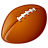# How to skip a blank field in a label expression?

481
2
03-06-2013 09:45 AMNew Contributor
Hello All,
I'm having difficulty running an label expression to skip a blank field.  Basically, I have 4 address fields plus a zip field.  I'm trying to write it so that the each address field is on its own line but if it's blank, skip the step.  I wrote it as a general function and got it to work in a message box but it won't work in the label expression shell and, of course, it doesn't debug.  I'm sure what I have isn't the most efficient but I only really dabble in code.
Thanks in advance for any help.
Here's what I have:

`Function FindLabel ( [ADR_LINE1], [ADR_LINE2], [ADR_LINE3], [ADR_LINE4], [ZIPCDE] )   Dim coll As New Collection   Dim ad1 As String, ad2 As String, ad3 As String, ad4 As String, labeltxt As String   Dim txt   ad1 = [ADR_LINE1]   ad2 = [ADR_LINE2]   ad3 = [ADR_LINE3]   ad4 = [ADR_LINE4]   coll.add ad1   coll.add ad2   coll.add ad3   coll.add ad4   For Each txt In coll     If txt <> "" Then       labeltxt = labeltxt + txt + vbNewLine      End If   Next txt   FindLabel = labeltxt + [ZIPCDE] End Function`
Tags (2)
1 Solution

Accepted SolutionsOccasional Contributor
Hi Ryan,
This should do the trick:

Dim strInput
if [ADR_LINE1] <> " " then
end if
if [ADR_LINE2] <> " " then
strInput = strInput & vbNewLine & [ADR_LINE2]
end if
if [ADR_LINE3] <> " " then
strInput = strInput & vbNewLine & [ADR_LINE3]
end if
if [ADR_LINE4] <> " " then
strInput = strInput & vbNewLine & [ADR_LINE4]
end if
if [ZIPCDE] <> " " then
strInput = strInput & vbNewLine & [ZIPCDE]
end if
FindLabel = strInput
End Function
2 RepliesOccasional Contributor
Hi Ryan,
This should do the trick:

Dim strInput
if [ADR_LINE1] <> " " then
end if
if [ADR_LINE2] <> " " then
strInput = strInput & vbNewLine & [ADR_LINE2]
end if
if [ADR_LINE3] <> " " then
strInput = strInput & vbNewLine & [ADR_LINE3]
end if
if [ADR_LINE4] <> " " then
strInput = strInput & vbNewLine & [ADR_LINE4]
end if
if [ZIPCDE] <> " " then
strInput = strInput & vbNewLine & [ZIPCDE]
end if
FindLabel = strInput
End FunctionNew Contributor
That did the trick.  Thank you very much, David.  🙂

I should have thought about coding each field instead of iterating a collection but I think I got tunnel vision since it worked in the VBA function

Cheers!